# GMAT Data Sufficiency Strategy: Test Cases

by , Nov 14, 2014If youre going to do a great job on Data Sufficiency, then youve got to know how to Test Cases. This strategy will help you on countless DS problems.

Try this GMATPrep problem from the free exams. Give yourself about 2 minutes. Go!

* On the number line, if the number k is to the left of the number t, is the product kt to the right of t?

(1) t < 0

(2) k < 1

If visualizing things helps you wrap your brain around the math (it certainly helps me), sketch out a number line: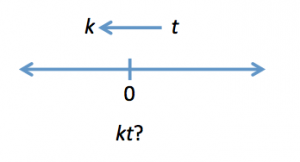k is somewhere to the left of t, but the two actual values could be anything. Both could be positive or both negative, or k could be negative and t positive. One of the two could even be zero.

The question asks whether kt is to the right of t. That is, is the product kt greater than t by itself?

There are a million possibilities for the values of k and t, so this question is what we call a theory question: are there certain characteristics of various numbers that would produce a consistent answer? Common characteristics tested on theory problems include positive, negative, zero, simple fractions, odds, evens, primesbasically, number properties.

(1) t < 0"

This problem appears to be testing positive and negative, since the statement specifies that one of the values must be negative. Test some real numbers, always making sure that t is negative.

Case #1: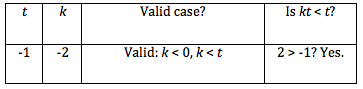Testing Cases involves three consistent steps:

First, choose numbers to test in the problem

Second, make sure that you have selected a valid case. All of the givens must be true using your selected numbers.

In this case, the answer is Yes. Now, your next strategy comes into play: try to prove the statement insufficient.

How? Ask yourself what numbers you could try that would give you the opposite answer. The first time, you got a Yes. Can you get a No

Case #2: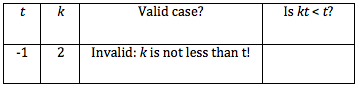Careful: this is where you might make a mistake. In trying to find the opposite case, you might try a mix of numbers that is invalid. Always make sure that you have a valid case before you actually try to answer the question. Discard case 2.

Case #3: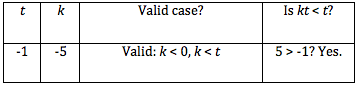Hmm. We got another Yes answer. What does this mean? If you cant come up with the opposite answer, see if you can understand why. According to this statement, t is always negative. Since k must be smaller than t, k will also always be negative.

The product kt, then, will be the product of two negative numbers, which is always positive. As a result, kt must always be larger than t, since kt is positive and t is negative.

Okay, statement (1) is sufficient. Cross off answers BCE and check out statement (2):

(2) k < 1

You know the drill. Test cases again!

Case #1: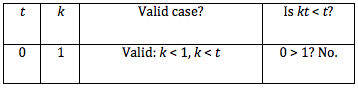Youve got a No answer. Try to find a Yes.

Case #2: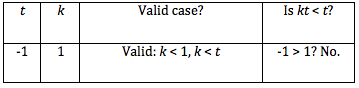Hmm. I got another No. What needs to happen to make kt > t? Remember what happened when you were testing statement (1): try making them both negative!

In fact, when youre testing statement (2), see whether any of the cases you already tested for statement (1) are still valid for statement (2). If so, you can save yourself some work. Ideally, the below would be your path for statement (2), not what I first showed above:

(2) k < 1

Case #1: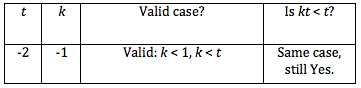All you have to do is make sure that the case is valid. If so, youve already done the math, so you know that the answer is the same (in this case, Yes).

Now, try to find your opposite answer: can you get a No?

Case #2: Try something I couldnt try before. k could be positive or even 0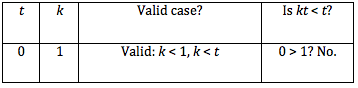Guess what? The technique can also work on some Problem Solving problems. Try it out on the following GMATPrep problem, then join me next week to discuss the answer:

* For which of the following functions f is f(x) = f(1 x) for all x?

(A) f(x) = 1 x

(B) f(x) = 1 [pmath]x^2[/pmath]

(C) f(x) = [pmath]x^2-(1-x)^2[/pmath]

(D) f(x) = [pmath]x^2(1-x)^2[/pmath]

(E) [pmath]f(x)=x/{1-x}[/pmath]

## Key Takeaways: Test Cases on Data Sufficiency

(1) When DS asks you a theory question, test cases. Theory questions allow multiple possible scenarios, or cases. Your goal is to see whether the given information provides a consistent answer.

(2) Specifically, try to disprove the statement: if you can find one Yes and one No answer, then youre done with that statement. You know its insufficient. If you keep trying different kinds of numbers but getting the same answer, see whether you can think through the theory to prove to yourself that the statement really does always work. (If you cant, but the numbers you try keep giving you one consistent answer, just go ahead and assume that the statement is sufficient. If youve made a mistake, you can learn from it later.)

* GMATPrep questions courtesy of the Graduate Management Admissions Council. Usage of this question does not imply endorsement by GMAC.